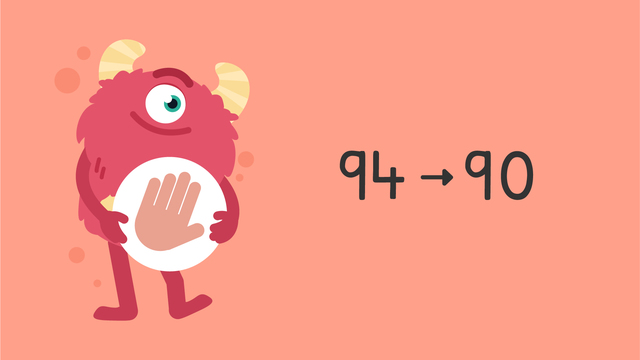# Rounding to the Nearest Ten — Let's Practice!Rating

Ø 5.0 / 2 ratings
The authorsTeam Digital
Rounding to the Nearest Ten — Let's Practice!
CCSS.MATH.CONTENT.3.NBT.A.1

## Basics on the topicRounding to the Nearest Ten — Let's Practice!

Today we are practicing rounding to the nearest ten with Razzi! This video contains examples to help you further practice and grow confident in this topic.

### TranscriptRounding to the Nearest Ten — Let's Practice!

Razzi says get these items ready, because today we're going to practice rounding to the nearest ten. It's time to begin! Round ninety-four to the nearest ten. Pause the video to work on the problem, and press play when you are ready to see the solution! Four is in the ones place, so round down. Ninety-four rounded to the nearest ten is ninety. Did you also get ninety? Let's tackle the next problem! Round thirty-six to the nearest ten. Pause the video to work on the problem, and press play when you are ready to see the solution! Six is in the ones place, so round up. Thirty-six rounded to the nearest ten is forty. Did you also get forty? Here is the next number! Round one hundred sixty-five to the nearest ten. Pause the video to work on the problem, and press play when you are ready to see the solution! Five is in the ones place, so round up. One hundred sixty-five rounded to the nearest ten is one hundred seventy. Did you also get one hundred seventy? Let's tackle the final problem! Round nine hundred twenty-one to the nearest ten. Pause the video to work on the problem, and press play when you are ready to see the solution! One is in the ones place, so round down. Nine hundred twenty-one to the nearest ten is nine hundred twenty. Did you also get nine hundred twenty? Razzi had so much fun practicing with you today! See you next time!

## Rounding to the Nearest Ten — Let's Practice! exercise

Would you like to apply the knowledge you’ve learned? You can review and practice it with the tasks for the video Rounding to the Nearest Ten — Let's Practice!.
• ### Round two digit numbers to the nearest 10.

Hints

Remember, when rounding to the nearest ten, look at the ones.

If the ones digit is 0, 1, 2, 3 or 4 - round down.

If the ones digit is 5, 6, 7, 8 or 9 - round up.

With the number 27, there is a 7 in the ones column. What do we do when the ones are 5, 6, 7, 8 or 9?

Solution

Here are the numbers rounded to the nearest 10.

• 49 = 50
• 35 = 40
• 23 = 20
• 54 = 50
• ### More rounding to 10.

Hints

Remember, when rounding to the nearest ten, look at the ones.

If the ones digit is 0, 1, 2, 3 or 4 - round down.

If the ones digit is 5, 6, 7, 8 or 9 - round up.

In this number, there is a 4 in the ones column. When we round this to the nearest ten, will we round up or down?

Solution

The numbers rounded to the nearest 10 are:

• 44 = 40
• 29 = 30
• 164 = 160
• 145 = 150
• ### Find the nearest 10.

Hints

Look at the digit in the ones column.

If this is 0, 1, 2, 3 or 4 round down.

If this is 5, 6, 7, 8 or 9 round up

Here is the number 45. In the ones column there is a 5. Would we round up or down?

Solution

The numbers rounded to the nearest 10 are:

• 75 = 80
• 93 = 90
• 62 = 60
• 15 = 20
• ### Round the numbers

Hints

Look at the digit in the ones column.

If this is 0, 1, 2, 3 or 4 round down.

If this is 5, 6, 7, 8 or 9 round up

In this number, what digit is in the ones column?

Try writing the number onto a place value chart to check which digit is in the ones column.

Solution

When each number is rounded to the nearest 10:

• 82 = 80
• 153 = 150
• 284 = 280
• 185 = 190
• 573 = 570
• ### Round to the nearest 10.

Hints

Look at the digit in the ones column.

If this is 0, 1, 2, 3 or 4 round down.

If this is 5, 6, 7, 8 or 9 round up.

In this number, the ones column has the number 4, so we round down to 50.

Solution

These numbers rounded to the nearest 10 are:

• 24 = 20
• 56 = 60
• 28 = 30
• 132 = 130
• ### Solve the problems by rounding.

Hints

Try looking at the numbers smaller than 460. How small can you go before the number would have to round down to 450 rather than up to 460?

Look at the ones in the number: 454. Would this number round up to 460 or round down to 450?

Solution

When rounding a number to the nearest 10 and reaching 460, the smallest possible number is 455 and the largest posssible number is 464.